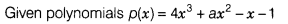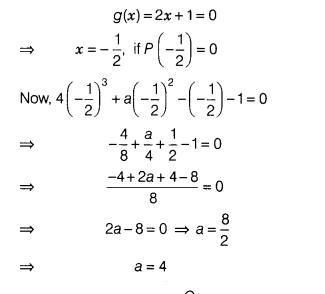# For what value of a is p(x) - 4x3 + ax2 - x -1 exactly divisible by g(x)=2x + 1?

For what value of a is p(x) - 4x3 + ax2 - x -1 exactly divisible by g(x)=2x + 1?and g(x)=2x+1
By the factor theorem,p(x) will be exactly divisible by